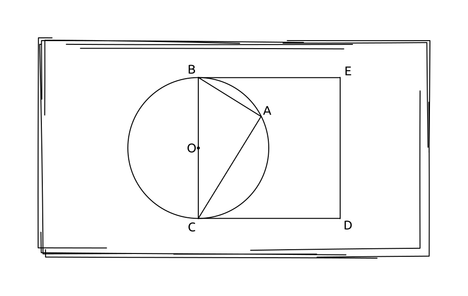# Ha Ha Haridowan007Replay of IUT 9th ICT Fes...
Limits 2s, 1.0 GB

Joker has finally created a master plan to defeat Batman. He has given Batman a geometric problem. Although, Batman is one of the world’s smartest men, he is afraid of geometric problems. But you are not, right? You can solve a geometric problem, can’t you?Here ABC is a circle with O as its center. BC is its diameter. So OB = OC. BCDE is a square so BC = CD = DE = EB and ∠EBC = ∠BCD = ∠CDE = ∠DEB = 90° or π/2 radians.

Now given the values of AB and AC, what is the area of square BCDE?

## Input

First line of the input is T (1 ≤ T ≤ 100). In each of next T line will describe T cases where you will be given two integers as the values of AB and AC (1 ≤ AB, AC ≤ 100).

## Output

For each test case print a line in “Case I: R” format where I is case number and R is the area of BCDE.

## Sample

InputOutput
3
1 1
3 4
4 3
Case 1: 2
Case 2: 25
Case 3: 25GeometryuDebug

### Submit